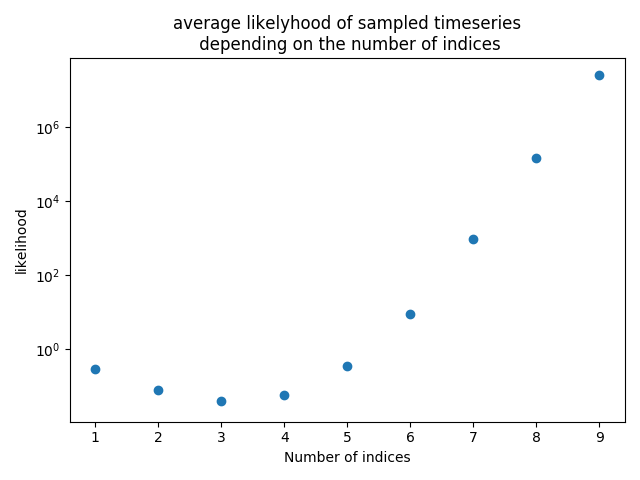# #StackBounty: #likelihood #gaussian-process Why does the likelihood of a random sample drawn from a Gaussian process change with the nu…

### Bounty: 100

I made some experiments to better understand how the likelihood of samples drawn from a Gaussian process change depending on the number of indices. For that purpose, I drew for different number of indices some samples, calculated their likelihood, and then took the mean of all samples (see python code at end of post). This resulted in following plot (careful: y-axis logarithmic):My assumption was that since the sample space increases with the number of indexes, the likelihood would get lower with increased number of indexes. Instead the we have a curve with a local minima at 3 (which is suspiciously close to $$e$$, but that might be pure coincidence) and then increases exponentially.

Why does this curve have the shape it has? Is there also a way to calculate this algebraic?

Python code that created the plot (simplified pseudo code below):

``````import numpy as np
import tensorflow_probability as tfp
import matplotlib.pyplot as plt
tfd = tfp.distributions
psd_kernels = tfp.math.psd_kernels

# The different number of indices we are going to evaluate
n_indices = range(1, 10)
# number of samples we are going to draw, higher == more stable
nsamples = 1000
# define some kernel, default values: amplitude=1., length_scale=1.
# initialize empty list for storing results
prob_mean_list = []
# run for each n_index
for n_index in n_indices:
# create x-axis indices
index_points = np.expand_dims(np.linspace(-1., 1., n_index), -1)
# initialize GP
gp = tfd.GaussianProcess(
kernel=kernel,
index_points=index_points)
# draw nsamples samples from the GP
samples = gp.sample(nsamples)
# calc the likelihood for each sample
prob = gp.prob(samples)
# take the mean of all likelihoods and store it for plotting
prob_mean = np.mean(prob.numpy())
prob_mean_list.append(prob_mean)

plt.scatter(n_indices, prob_mean_list)
plt.yscale('log')
plt.xlabel("Number of indices")
plt.ylabel("likelihood")
plt.title("average likelihood of sampled timeseriesn depending on the number of indices")
plt.show()
``````

Pseudo code of the python code:

``````for each number of indices n (1 to 9):
draw 1000 samples from a gaussian process with n indexes (each sample is a vector of lenght n). It uses an exponentiated quadratic kernel with amplitude = 1 and length scale = 1.
calculate the likelihood of obtaining each sample
take the mean of all likelyhoods
plot this mean value
``````

Get this bounty!!!

This site uses Akismet to reduce spam. Learn how your comment data is processed.